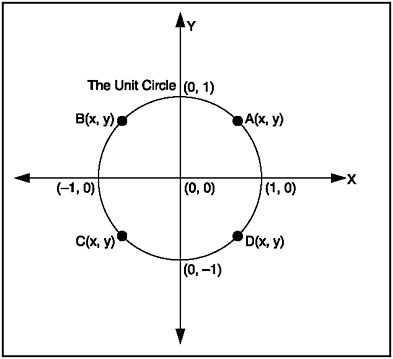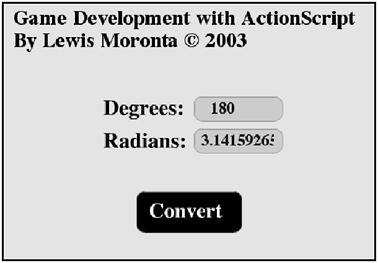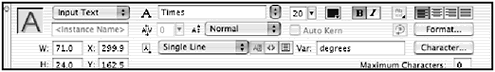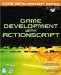[ LiB ]

If you were to measure a circle along its circumference, the unit measure you would use would be a radian. In other words, a radian is the unit measure along the circumference of a circle, the unit measure of an arc. Radians are also the unit values that the trigonometric functions in ActionScript accept. Any positive degree from 0 to 359 can represent an individual point on the circumference. See Figure 11.5.

##### Figure 11.5. Points, in radians, along the circumferenceMost of us were raised to think of a circle as 360 degrees, which makes working with radians seem counter-intuitive. Fortunately, there is a way to work entirely with degrees while still passing ActionScript's trig functions their parameters in the form of radians. To do this, all we need to do is convert our degree values to radians just before calling these functions. How do you convert a degree to a radian? Check out the following formula:

Pi is that magical number that keeps popping up in all places; it can be rounded to ~3.1415 as an accurate measure.

NOTE

TIP

If the objects are grouped on the stage, you can ungroup them using the Modify menu.You can also double- click on the group to edit it.You can always go back to the Timeline by pressing on the stage controls.

I've written a program that converts degrees to radians for you. You can find it on the CD as GDA_PROG11.2.fla. Figure 11.6 shows this demo.

##### Figure 11.6. Converting degrees to radiansOpen the FLA file and notice the properties, in the Properties panel, for the first textbox. Check out Figure 11.7 and see what options I selected.

##### Figure 11.7. Adjusting input textbox propertiesYou can see that I have selected Input Text from the drop-down menu and assigned degrees in the input box labeled Var:. This causes the degrees variable to update itself with the contents of the textboxespecially when the user types something in.

The textbox over the button on the stage is a normal dynamic box with a variable attached. The rest of the script lies within the buttoncheck out the following listing.

` // Game Development with ActionScript // By Lewis Moronta (c) 2003 // This program converts degrees // to radians. on (release) {   // Convert to Integer   intDeg = parseInt(degrees);   // Get the value of one radian   oneRad = Math.PI/180;   // Finally convert one radian to how many degrees   radians = oneRad * intDeg; } `

This is the listing in the Convert buttonall of the calculations happen in this button and the calculations start when the user releases from clicking it.

` on (release) { `

As degrees is the variable attached to the input box, its content is in string format. As I need this value in a formula, I need to convert the value to a number so I can actually operate on it. This is where parseInt comes in. This command converts a string to its numerical equivalent.

` intDeg = parseInt(degrees); `

In order to get the value of one radian, you have to divide the same amount of radians by the equivalent value in degrees to get 1 radian. I used Pi and 180 degrees to get my value.

` // Get the value of one radian oneRad = Math.PI/180; `

According to the formula above, if you multiply onRad by intDeg , you should get the value of radians that you want. This is exactly what I did:

` // Finally convert one radian to how many degrees radians = oneRad * intDeg; `

And that's what it takes to convert degrees to radians in Flash.

 [ LiB ]Game Development with ActionScript
ISBN: 1592001106
EAN: 2147483647
Year: 2004
Pages: 162
Authors: Lewis Moronta